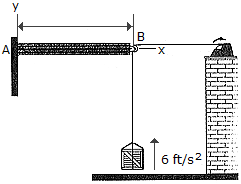# Engineering Mechanics - KOP: Force and Acceleration - Discussion

### Discussion :: KOP: Force and Acceleration - General Questions (Q.No.2)

2.The 30-lb crate is being hoisted upward with a constant acceleration of 6 ft/s2. If the uniform beam AB has a weight of 200 lb, determine the components of reaction at A. Neglect the size and mass of the pulley at B.

 [A]. Ax = -48.3 lb, Ay = 248.3 lb, MA = 258 lb-ft CCW [B]. Ax = -48.3 lb, Ay = 248.3 lb, MA = 741 lb-ft CCW [C]. Ax = -35.6 lb, Ay = 236 lb, MA = 678 lb-ft CCW [D]. Ax = -30.0 lb, Ay = 230 lb, MA = 650 lb-ft CCW

Explanation:

No answer description available for this question.